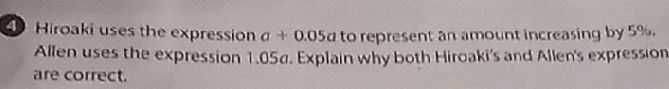### Still have math questions?4) Hiroaki uses the expression $$a + 0.05 a$$ to represent an amount increasing by $$5 \%$$ . Allen uses the expression $$1.05 a$$ . Explain why both Hiroaki's and Allen's expression are correct.
a+0.05a=1.05a=105$$\%$$a